# Kronecker theorem

Given,, and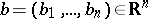; then for anythere exist integers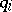,, and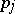,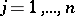, such that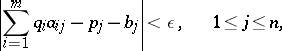if and only if for any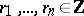such thatthe numberis also an integer. This theorem was first proved in 1884 by L. Kronecker (see ).

Kronecker's theorem is a special case of the following theorem , which describes the closure of the subgroup of the torusgenerated by the elements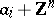,: The closure is precisely the set of all classessuch that, for any numberswith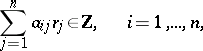one has also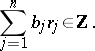(Cf. .) Under the assumptions of Kronecker's theorem, this closure is simply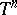. This means that the subgroup of all elements of the form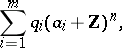where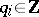, is dense in, while the subgroup of vectorswhere, is dense in. Kronecker's theorem can be derived from the duality theory for commutative topological groups (cf. Topological group), .

In the case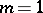, Kronecker's theorem becomes the following proposition: A class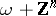, where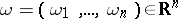, generatesas a topological group if and only if the numbers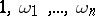are linearly independent over the fieldof rational numbers. In particular, the torusas a topological group is monothetic, i.e. is generated by a single element.